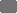# gms | German Medical Science

## GMDS 2015: 60. Jahrestagung der Deutschen Gesellschaft für Medizinische Informatik, Biometrie und Epidemiologie e. V. (GMDS)

Deutsche Gesellschaft für Medizinische Informatik, Biometrie und Epidemiologie

06.09. - 09.09.2015, Krefeld

## Confounder-Equivalence in DAG-based Confounder-Selection – Results of a Simulation Study

Meeting Abstract

### Search Medline for

•Frauke Hennig - IUF-Leibniz Institut für umweltmedizinische Froschung, Deutschland; IMIBE- Institute for Medical Informatics, Biometry and EpidemiologyUniversity Hospital, University Duisburg-Essen, Deutschland; Faculty of Statistics, TU Dortmund University, Dortmund, Deutschland
•Barbara Hoffmann - IUF-Leibniz Research Institute for Environmental Medicine, Düsseldorf, Deutschland; Heinrich Heine University of Düsseldorf, Medical Faculty, Deanery of Medicine, Düsseldorf, Deutschland
•Katja Ickstadt - Faculty of Statistics, TU Dortmund University, Dortmund, Deutschland

GMDS 2015. 60. Jahrestagung der Deutschen Gesellschaft für Medizinische Informatik, Biometrie und Epidemiologie e.V. (GMDS). Krefeld, 06.-09.09.2015. Düsseldorf: German Medical Science GMS Publishing House; 2015. DocAbstr. 164

Published: August 27, 2015

### Text

Introduction: Adjustment for confounding variables in observational studies is still the most essential task faced by epidemiological researchers. In 1999 Greenland et al. proposed the DAG (directed acyclic graph)-approach as a non-parametric tool for confounder identification in epidemiology. This method assumes that, given an underlying conditional dependency structure of data, including exposure (X), outcome (Y), and potential confounding variables (C1,….Ck), visualized as a DAG, there exists a sufficient set S of variables that one needs to adjust for in order to get an unbiased exposure effect estimate of X on Y , . However, sometimes a given structure predicts equality of two or more sufficient adjustment sets, although when applied to data such equality, i.e. equal bias-reduction, is not found. We aim to evaluate a strategy to rank multiple sets S according to their bias-reducing potential by means of confounder (c)-equivalence criteria  and conditional dependencies among variables.

Methods: We simulated multivariate normally distributed data, consisting of 5000 observations and variables X (=exposure), Y (=outcome) and 2 confounding variables (C1, C2), according to an a priori DAG-structure, resulting into two equivalent sufficient adjustment sets. Strengths of causal relation (beta-coefficients) were randomly chosen from a uniform distribution over 0.5 to 2, except the beta-coefficient for the causal relation between X and Y, which was set to 1. Error variances were set to 1. First, we learned the structure of the simulated data using the hill-climbing greedy search algorithm and evaluated assumed conditional independencies in terms of strength and significance. If a conditional independency statement did not hold, the DAG was updated. Secondly, we evaluated sufficient adjustment sets and in case of multiple sufficient adjustments set we proved c-equivalence by formal theorems . In terms of no c-equivalence we ranked multiple adjustment sets Si by their bias-introducing potential, calculated as the sum of conditional correlations cor((Y,Si)|X)+cor((X,Si)|Y) (SCC) and adjusted for Si, that maximizes SCC.

Results: In this first simulation study, including X,Y and 2 confounding variables (C1, C2), the true DAG, promising c-equivalence of \'7bC1\'7d and \'7bC2\'7d, was found in 98.7% and corresponding conditional independency statements was held in 99.9%. However conditions for c-equivalence were not held in 80 of 987 cases and the bias-reducing potential of \'7bC1\'7d and \'7bC2\'7d differed markedly with a mean squared error (MSE) of 0.0269 and 0.0098. In 907 cases, where criteria for c-equivalence held, however, a different bias-reducing potential was still observed with a MSE of 0.0151 and 0.0093. Overall bias was 0.0161 (0.0151 if c-equivalent, 0.0269 if not). After applying the strategy of adjusting for the set that maximized SCC, whenever c-equivalence was not reached, we were able to reduce bias to a certain degree (MSE=0.0121). When we applied this strategy to all cases of multiple sufficient sets, bias was reduced to a MSE of 0.0089.

Discussion: Results from our first simulation scenario show, that c-equivalence among two proposed sufficient adjustment sets was not observed given the data, independent of the validity of formal criteria of c-equivalence. Ranking multiple adjustment sets according to their bias-introducing potential, calculated as the sum of conditional correlations between Si and Y given X and vice versa, and adjusting for the set Si maximizing this sum, bias was reduced remarkably in all cases. So, even if c-equivalence holds one set seems to have a higher bias-reducing potential than the other. It was straightforward to evaluate the bias-introducing potential when multiple sets included only one variable, but might be more challenging in multiple-variable cases. In further steps we thus want to adapt this strategy to multiple-variable cases applying further simulations.

### Literatur

1.
Pearl J. Causal diagrams for empirical research. Biometrica. 1995;(82):669-710.
2.
Pearl J, Paz A. Confounding Equivalence in Causal Inference. Journal of Causal Inference. 2014; 2(October):75–93. DOI: 10.1515/jci-2013-00203.
Greenland S, Pearl J, Robins JM. Causal Diagrams for Epidemiological Research. Epidemiology. 1999;(10):37–48.